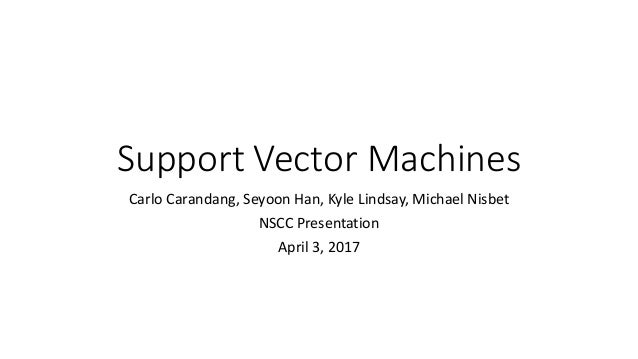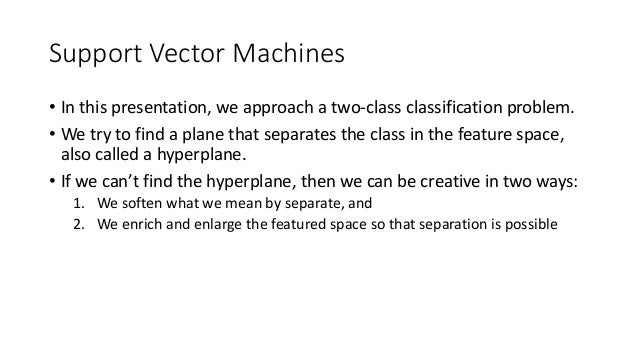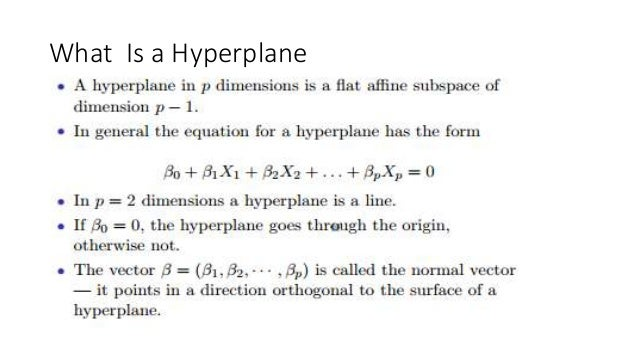Successfully reported this slideshow.Upcoming SlideShare
×

of1

Share

# Support Vector Machines- SVM

In this presentation, we approach a two-class classification problem. We try to find a plane that separates the class in the feature space, also called a hyperplane. If we can't find a hyperplane, then we can be creative in two ways: 1) We soften what we mean by separate, and 2) We enrich and enlarge the featured space so that separation is possible.

See all

### Support Vector Machines- SVM

1. 1. Support Vector Machines Carlo Carandang, Seyoon Han, Kyle Lindsay, Michael Nisbet NSCC Presentation April 3, 2017
2. 2. Support Vector Machines • In this presentation, we approach a two-class classification problem. • We try to find a plane that separates the class in the feature space, also called a hyperplane. • If we can’t find the hyperplane, then we can be creative in two ways: 1. We soften what we mean by separate, and 2. We enrich and enlarge the featured space so that separation is possible
3. 3. What Is a Hyperplane
4. 4. Hyperplane in 2 Dimensions
5. 5. Separating Hyperplanes
6. 6. Maximal Margin Classifier *This can be rephrased as a convex quadratic program and solved efficiently. The function svm() in package e1071 solves this problem efficiently.
7. 7. The data on the left are not separable by a linear boundary. This is often the case, unless N < p. Non-separable Data
8. 8. Noisy Data Sometimes the data are separable, but noisy. This can lead to a poor solution for the maximal-margin classifier. The support vector classifier maximizes a soft margin.
9. 9. Support Vector Classifier
10. 10. C is a Regularization parameter
11. 11. Linear boundary can fail Sometimes a linear boundary simply won’t work, no matter what value of C. The example is on the left is the case. What to do?
12. 12. Support Vector Classifier and Non-Linear Class Boundaries • The support vector classifier is a natural approach for classification in the two-class setting, if the boundary between the two classes is linear • However, in practice we are sometimes faced with non-linear class boundaries • In this case, the soft margin is not going to help
13. 13. Feature Expansion- Linear Regression • In Chapter 7, we saw that linear regression suffers when there is a non-linear relationship between predictors (independent variables) and the outcome measures (dependent variables) • The solution is enlarging the feature space using functions of the predictors, such as quadratics and cubic terms, in order to address this non-linearity: • ax2 + bx + c = 0 (quadratic) • ax3 + bx2 + cx + d = 0 (cubic)
14. 14. Feature Expansion- Support Vector Classifier • So for Support Vector Classifier, we can address non-linear boundaries between classes in a similar way, by enlarging the feature space using quadratic, cubic, and higher-order polynomial functions of the predictors • For instance, rather than fitting a support vector classifier using p features: X1, X2, . . . , Xp • We can instead fit a support vector classifier using 2p features: X1, X1 2, X2, X2 2, . . . , Xp, Xp 2
15. 15. Support Vector Machine • This results in non-linear decision boundaries in the original space • Here is a cubic polynomial (X3)- degree 3 • Decision boundary split in two • Conic section of a cubic polynomial • This feature expansion of the support vector classifier known as the SUPPORT VECTOR MACHINE • Β0 + β1X1 + β2X2 + β3X1 2 + β4X2 2 + β5X1X2 + β6X1 3 + β7X2 3 + β8X1X 2 2 + β9X1 2X2 = 0
16. 16. Non-Linearities and Kernels • Polynomials (especially high-dimensional ones) get wild rather fast • In regression, we don’t like doing polynomial regression with degree larger than 3 • In support-vector classifiers, there is a more elegant and controlled way to introduce nonlinearities— through the use of kernels • Before we discuss these, we must understand the role of inner products in support-vector classifiers
17. 17. Inner Products and Support Vectors • If we can compute the inner products between all pairs of observations and if we can also compute the inner products between all the training observations and a new test point, then we can both fit the support vector machine and evaluate the function
18. 18. Support Vectors
19. 19. Support Vectors • Support vectors (support points) are the alphas that are not zero • If a point is not a support point, then it is on the right side of the margin, and it does not affect the direction of the decision boundary • The alphas are assigning weights to the data points, and the ones that are zero (right side of the margin) have no bearing on the solution, while the data points that are not zero (support points) affect the solution
20. 20. Kernels and Support Vector Machines • Computing the inner products between observations can be quite abstract • Kernel functions can help and do this abstract math and compute the inner products for us:
21. 21. Kernels and Support Vector Machines • We don't need to actually visit the feature space because this kernel function will compute those inner products- sort of like magic • You've got a kernel function the computes this inner product in this very high dimensional space • The support vector machine (SVM) is an extension of the support vector classifier that results from enlarging the feature space in a specific way using kernels
22. 22. Radial Kernel • Radial kernels are very popular • One of the most popular kernels that's used for non-linear support vector machines • With feature expansion of support vector classifier, you'd run into trouble raising power to 1,000,000 • But with a polynomial kernel in SVMs, you could get away with that because of all the squishing of the dimensions to zero
23. 23. Reference: Stanford University HumanitiesScience StatLearning: https://lagunita.stanford.edu/c4x/HumanitiesSciences/StatLearning/as set/svm-handout.pdf
•May. 5, 2019

Total views

539

On Slideshare

0

From embeds

0

Number of embeds

0

9

Shares

0